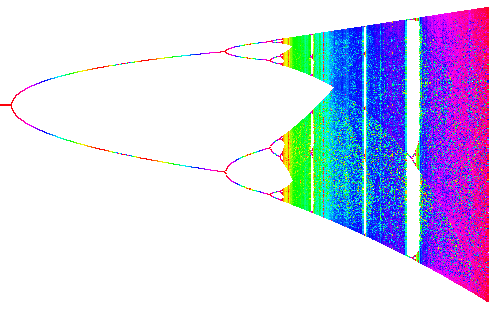# Periodic points of period 2,3If you move the mouse over the bifurcation diagram to the left the parameter c in the logistic map f(x) = c x (1-x) changes from c=0 to c=4. You see to the right the graphs of f(x) f(f(x)) and f(f(f(x)). If the graph y=f(f(f(x)) intersects the diagonal y=x at a place where y=f(x) does not intersect the y=x, we have a periodic point of prime period 3.